StatLect

Relations among modes of convergence

In the previous lectures, we have introduced several notions of convergence of a sequence of random variables (also called modes of convergence). There are several relations among the various modes of convergence, which are discussed below and are summarized by the following diagram (an arrow denotes implication in the arrow's direction):.Almost sure convergence implies convergence in probability

If a sequence of random variablesconverges almost surely to a random variable, thenalso converges in probability to.

Proof

See, e.g., Resnick (1999).

Convergence in probability implies convergence in distribution

If a sequence of random variablesconverges in probability to a random variable, thenalso converges in distribution to.

Proof

See, for example, Resnick (1999).

Almost sure convergence implies convergence in distribution

If a sequence of random variablesconverges almost surely to a random variable, thenalso converges in distribution to.

Proof

This is obtained putting together the previous relations (almost sure convergence implies convergence in probability, which in turn implies convergence in distribution).

Mean square convergence implies convergence in probability

If a sequence of random variablesconverges in mean square to a random variable, thenalso converges in probability to.

Proof

We can apply Markov inequality to a generic term of the sequence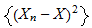:for any strictly positive real number. Taking the square root of both sides of the left-hand inequality, we obtain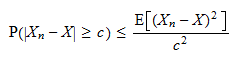Taking limits on both sides, we get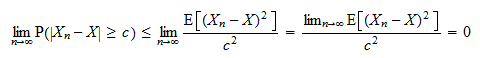where we have used the fact that, by the very definition of convergence in mean square,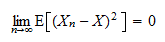Since, by the definition of probability, it must be that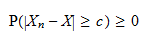then it must be that also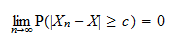Note that this holds for any arbitrarily small. By the definition of convergence in probability, this means thatconverges in probability to(if you are wondering about strict and weak inequalities here and in the definition of convergence in probability, note thatimplies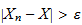for any strictly positive).

Mean square convergence implies convergence in distribution

If a sequence of random variablesconverges in mean square to a random variable, thenalso converges in distribution to.

Proof

This is obtained putting together the previous relations (mean square convergence implies convergence in probability, which in turn implies convergence in distribution).

References

Resnick, S. I. (1999) A probability path, Birkhauser.

The book

Most of the learning materials found on this website are now available in a traditional textbook format.

Glossary entries
Share